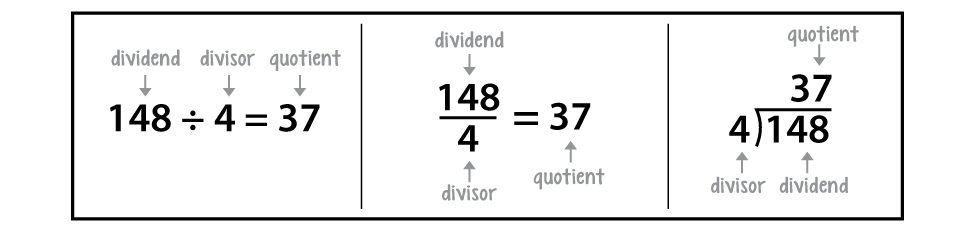# Lesson 3: Division Review

## Getting Started

Now that you've practiced multiplying whole and decimal numbers, you're ready to review division as well. You probably remember that division is the opposite of multiplication. For example, 3 × 2 = 6, and 6 ÷ 2 = 3. Division can be represented in several ways:Each of these can be read as twelve divided by three.

In a division problem, the number that is being divided is called the dividend and the number that does the dividing is called the divisor. The answer is called the quotient.Just as with the other operations, there is an algorithm for dividing whole numbers that can also be used for dividing decimal numbers with just an extra step or two added.

### Stuff You Need

• 3-hole drilled card stock (kit)
• colored pencils
• construction paper (kit)
• glue or glue stick
• Interactive Notebook
• marker
• scissors

• How is division like repeated subtraction?
• How can using divisibility rules be used when dividing larger dividends?
• What are some ways to interpret remainders in division problems?
• In what ways might a remainder affect the answer to a word problem?

### Things to Know

• Division is the opposite of multiplication. For example, 3 × 2 = 6, and 6 ÷ 2 = 3.
• Division is repeated subtraction. In other words, 8 ÷ 4 means 8 − 4 = 4 − 4 = 0. Four can be subtracted from eight two times.
• In a division problem, the number being divided is called the dividend and the number that does the dividing is called the divisor. In the problem 6 ÷ 2 = 3, the number 6 is the dividend that is being divided by the divisor, which is 2. The answer to a division problem is called the quotient.
• If one number is divisible by another, it means that the quotient has no remainders. For example, 10 is divisible by 5 but not by 3.
• Remainders in division word problems can be interpreted in different ways. The remainder may need to be included, discarded, or used to round an answer up by 1. Using decimals and zeros can allow division to continue so that no remainder is needed.

### Skills

• Fluently divide multi-digit numbers using the standard algorithm
• Fluently divide multi-digit decimals using the standard algorithm
• Solve real-world and mathematical problems involving the four operations with rational numbers

### Introducing the Lesson

In this lesson, your child will review and practice skills for long division involving whole and decimal numbers. He will learn rules for divisibility that will help him more quickly and efficiently divide numbers, and he will develop strategies for interpreting how to deal with remainders in division problems.